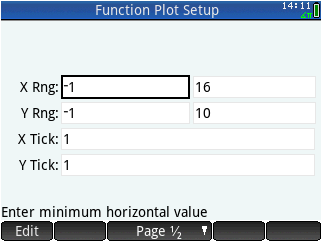plotting time discrete data - Printable Version +- HP Forums (https://www.hpmuseum.org/forum) +-- Forum: HP Calculators (and very old HP Computers) (/forum-3.html) +--- Forum: HP Prime (/forum-5.html) +--- Thread: plotting time discrete data (/thread-4207.html) plotting time discrete data - leprechaun - 06-23-2015 12:18 PM Hi everybody! I have a vector of time-discrete data. How do I plot a one dimensional vector? Is there an app that does the Job or a smart way? Either simply dots at the sampled Points or a polyline (implied polyline would be great anyway)? say M1:=[0,1,2,3,4,0,1,2,3,4,0,1,2,3,4]; is a sampled sawtooth where the index into the vector is supposed to be my sample index. No absolute time needed, just implied tn. RE: plotting time discrete data - Didier Lachieze - 06-23-2015 02:02 PM You can use the "Statistics 1Var" app, after selecting it you need to move your data to the D1 list: [attachment=2200] then in the Symb view select Line as the type of Plot: [attachment=2201] and with Plot you can view your data: [attachment=2202] RE: plotting time discrete data - leprechaun - 06-23-2015 02:10 PM I will present some results of my work. Not nearly satisfactional.... I decided to make a polyline(M) function that accepts a 1-dimensional vector as argument. It should use LINE_P() for plotting a polyline. That sounds nice, but I have the Feeling that i am stuck. For my general understanding: LINE_P accepts a Vector of Points, i.e. vectors with 2 entries for the 2-dimensional Problems and a Vector of indices into the above buffer that define line segments. Similar to a vbo I think? Help or an example on LINE_P with Point lists would be great! second problem: when trying to convert my 1-dim vector into a points array I fail. Code: ``` EXPORT polyline(data) BEGIN LOCAL v_data := MAKEMAT(I,length(data),2); v_data(2):=TRN(data); //How to assign? What should the Points Array look like? //LOCAL l_data:={{1,2},{2,3},{3,4},{4,5},{5,6}}; //How can I build such a vector in Dependance of length(data)? And row/column? Which format //Will deal with that later....... //RECT(); //LINE_P(v_data,l_data); //FREEZE; RETURN v_data; //Output for debugging END;``` How can assign a column in v_data? I realize that I am totally confused with row- and column vectos AND I do not know the best way to manipulate either with my prime. Thanks a lot for your help. It will lead to a working function, hopefully! RE: plotting time discrete data - leprechaun - 06-23-2015 02:11 PM Thanks Didier! However maybe you can help me getting my polyline() function working? It is a good way to learn. RE: plotting time discrete data - Didier Lachieze - 06-23-2015 03:32 PM (06-23-2015 02:11 PM)leprechaun Wrote:  Thanks Didier! However maybe you can help me getting my polyline() function working? It is a good way to learn. Here is a very rough implementation of the polyline() function, you'll have to adjust the pixel mapping to your needs: Code: ```EXPORT polyline(data) BEGIN   LOCAL x_max, y_max;   LOCAL L_Points, L_Lines;   x_max:= length(data); y_max:=MAX(data);   L_Points := MAKELIST({IP(I*300/x_max)+10, 220-IP(data(I)*220/y_max)+10},I,1,x_max);   L_Lines := MAKELIST({I,I+1},I,1, x_max-1);   RECT();   LINE_P(10,230,320,230); LINE_P(10,10,10,230);   // Draw X & Y Axis   LINE_P(L_Points,L_Lines,[[1,0],[0,1]]);   FREEZE;   RETURN L_Points; //Output for debugging END;``` RE: plotting time discrete data - Marcus von Cube - 06-24-2015 12:19 PM Here is another rather simple way using the Function app: